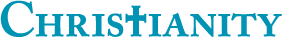## What percentage of my answers are accepted?

0

(click on this box to dismiss)

Returns the total number of answers, the number of accepted answers, and what percentage of answers are accepted.Q&A for committed Christians, experts in Christianity and those interested in learning more

``````-- What percentage of my answers are accepted?
-- Returns the total number of answers, the number of accepted answers, and what percentage of answers are accepted.

select
sum(case when q.AcceptedAnswerId IS NULL then 0 else 1 end) as [Accepted Answers],
round(sum(case when q.AcceptedAnswerId IS NULL then 0 else 1 end) * 100.0 / count(a.Id), 3) as [Percentage Accepted]
from
Posts a
left join
Posts qHold tight while we fetch your results